# augmenters.imgcorruptlike¶

## GaussianNoise¶

Wrapper around `gaussian_noise()`.

Note

This augmenter only affects images. Other data is not changed.

API link: `GaussianNoise`

The image below visualizes severities `1` to `5` – one severity per row: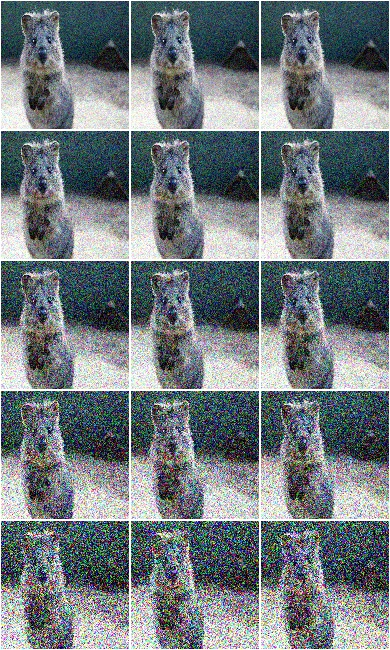Example. Create an augmenter around `gaussian_noise()`. Apply it to images using e.g. `aug(images=[image1, image2, ...])`:

```import imgaug.augmenters as iaa
aug = iaa.imgcorruptlike.GaussianNoise(severity=2)
```

## ShotNoise¶

Wrapper around `shot_noise()`.

Note

This augmenter only affects images. Other data is not changed.

API link: `ShotNoise`

The image below visualizes severities `1` to `5` – one severity per row: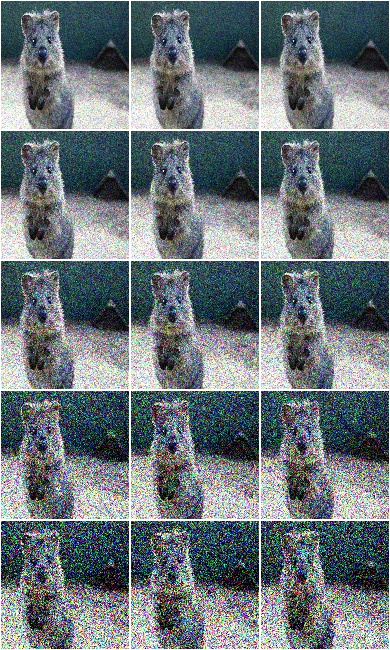Example. Create an augmenter around `shot_noise()`. Apply it to images using e.g. `aug(images=[image1, image2, ...])`:

```import imgaug.augmenters as iaa
aug = iaa.imgcorruptlike.ShotNoise(severity=2)
```

## ImpulseNoise¶

Wrapper around `impulse_noise()`.

Note

This augmenter only affects images. Other data is not changed.

API link: `ImpulseNoise`

The image below visualizes severities `1` to `5` – one severity per row: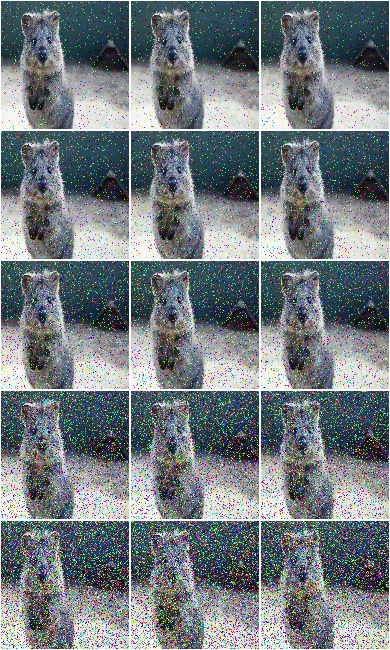Example. Create an augmenter around `impulse_noise()`. Apply it to images using e.g. `aug(images=[image1, image2, ...])`:

```import imgaug.augmenters as iaa
aug = iaa.imgcorruptlike.ImpulseNoise(severity=2)
```

## SpeckleNoise¶

Wrapper around `speckle_noise()`.

Note

This augmenter only affects images. Other data is not changed.

API link: `SpeckleNoise`

The image below visualizes severities `1` to `5` – one severity per row: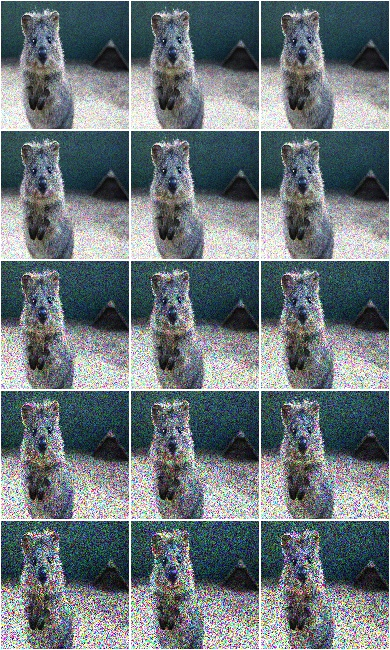Example. Create an augmenter around `speckle_noise()`. Apply it to images using e.g. `aug(images=[image1, image2, ...])`:

```import imgaug.augmenters as iaa
aug = iaa.imgcorruptlike.SpeckleNoise(severity=2)
```

## GaussianBlur¶

Wrapper around `gaussian_blur()`.

Note

This augmenter only affects images. Other data is not changed.

API link: `GaussianBlur`

The image below visualizes severities `1` to `5` – one severity per row: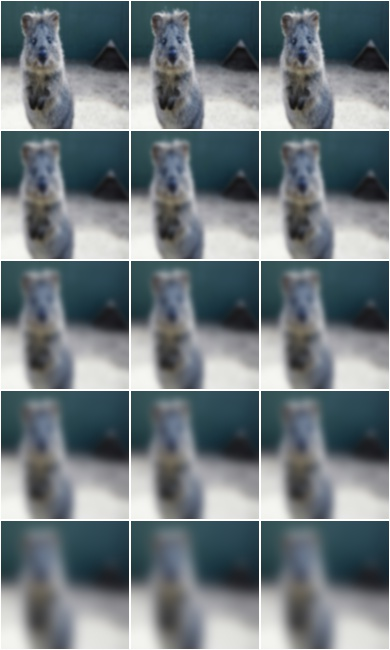Example. Create an augmenter around `gaussian_blur()`. Apply it to images using e.g. `aug(images=[image1, image2, ...])`:

```import imgaug.augmenters as iaa
aug = iaa.imgcorruptlike.GaussianBlur(severity=2)
```

## GlassBlur¶

Wrapper around `glass_blur()`.

Note

This augmenter only affects images. Other data is not changed.

API link: `GlassBlur`

The image below visualizes severities `1` to `5` – one severity per row: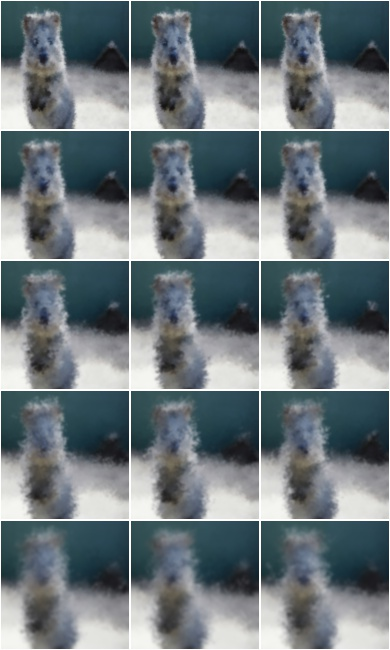Example. Create an augmenter around `glass_blur()`. Apply it to images using e.g. `aug(images=[image1, image2, ...])`:

```import imgaug.augmenters as iaa
aug = iaa.imgcorruptlike.GlassBlur(severity=2)
```

## DefocusBlur¶

Wrapper around `defocus_blur()`.

Note

This augmenter only affects images. Other data is not changed.

API link: `DefocusBlur`

The image below visualizes severities `1` to `5` – one severity per row: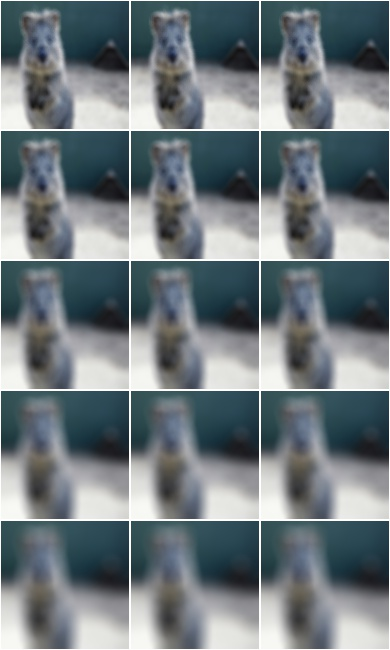Example. Create an augmenter around `defocus_blur()`. Apply it to images using e.g. `aug(images=[image1, image2, ...])`:

```import imgaug.augmenters as iaa
aug = iaa.imgcorruptlike.DefocusBlur(severity=2)
```

## MotionBlur¶

Wrapper around `motion_blur()`.

Note

This augmenter only affects images. Other data is not changed.

API link: `MotionBlur`

The image below visualizes severities `1` to `5` – one severity per row: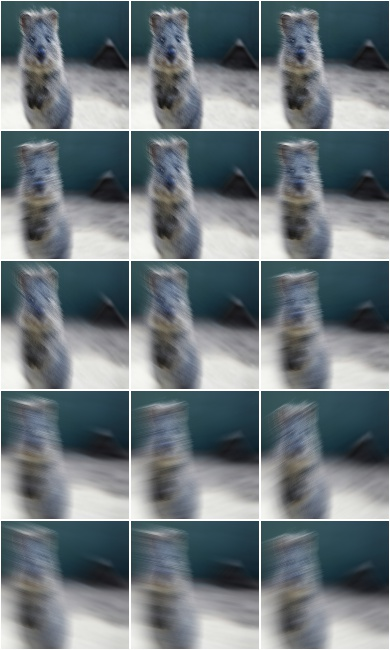Example. Create an augmenter around `motion_blur()`. Apply it to images using e.g. `aug(images=[image1, image2, ...])`:

```import imgaug.augmenters as iaa
aug = iaa.imgcorruptlike.MotionBlur(severity=2)
```

## ZoomBlur¶

Wrapper around `zoom_blur()`.

Note

This augmenter only affects images. Other data is not changed.

API link: `ZoomBlur`

The image below visualizes severities `1` to `5` – one severity per row: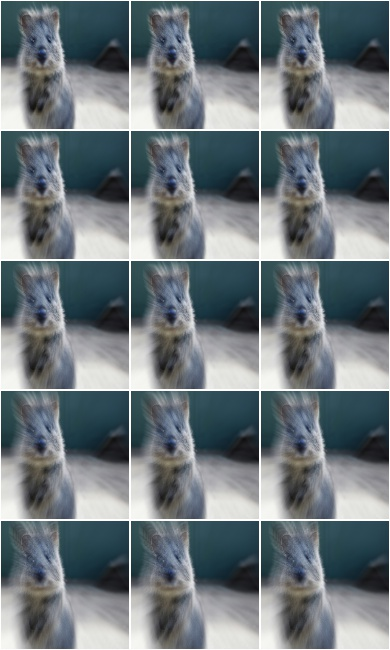Example. Create an augmenter around `zoom_blur()`. Apply it to images using e.g. `aug(images=[image1, image2, ...])`:

```import imgaug.augmenters as iaa
aug = iaa.imgcorruptlike.ZoomBlur(severity=2)
```

## Fog¶

Wrapper around `fog()`.

Note

This augmenter only affects images. Other data is not changed.

API link: `Fog`

The image below visualizes severities `1` to `5` – one severity per row: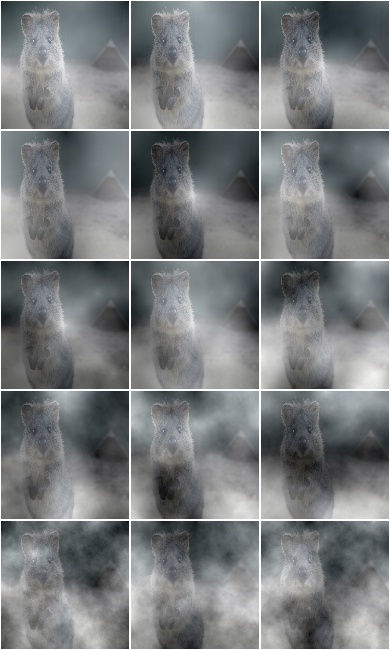Example. Create an augmenter around `fog()`. Apply it to images using e.g. `aug(images=[image1, image2, ...])`:

```import imgaug.augmenters as iaa
aug = iaa.imgcorruptlike.Fog(severity=2)
```

## Frost¶

Wrapper around `frost()`.

Note

This augmenter only affects images. Other data is not changed.

API link: `Frost`

The image below visualizes severities `1` to `5` – one severity per row: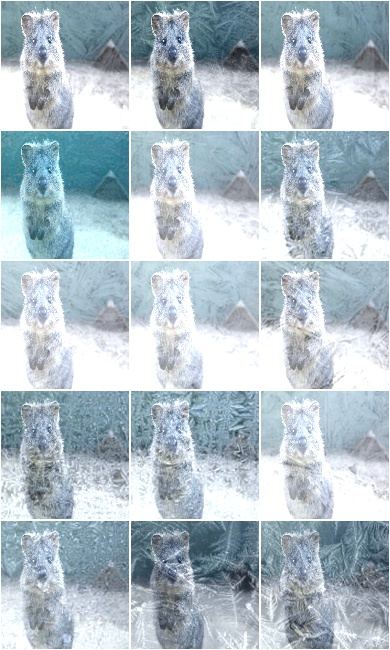Example. Create an augmenter around `frost()`. Apply it to images using e.g. `aug(images=[image1, image2, ...])`:

```import imgaug.augmenters as iaa
aug = iaa.imgcorruptlike.Frost(severity=2)
```

## Snow¶

Wrapper around `snow()`.

Note

This augmenter only affects images. Other data is not changed.

API link: `Snow`

The image below visualizes severities `1` to `5` – one severity per row: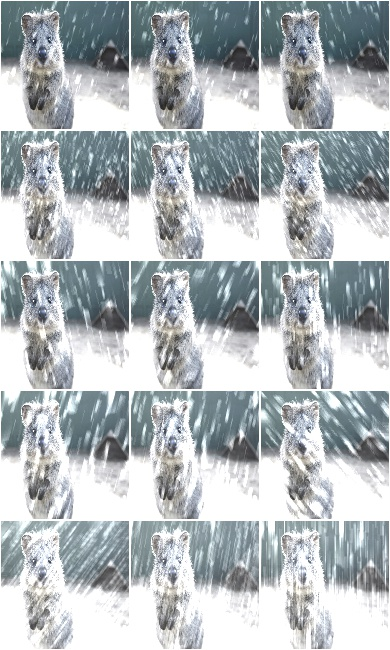Example. Create an augmenter around `snow()`. Apply it to images using e.g. `aug(images=[image1, image2, ...])`:

```import imgaug.augmenters as iaa
aug = iaa.imgcorruptlike.Snow(severity=2)
```

## Spatter¶

Wrapper around `spatter()`.

Note

This augmenter only affects images. Other data is not changed.

API link: `Spatter`

The image below visualizes severities `1` to `5` – one severity per row: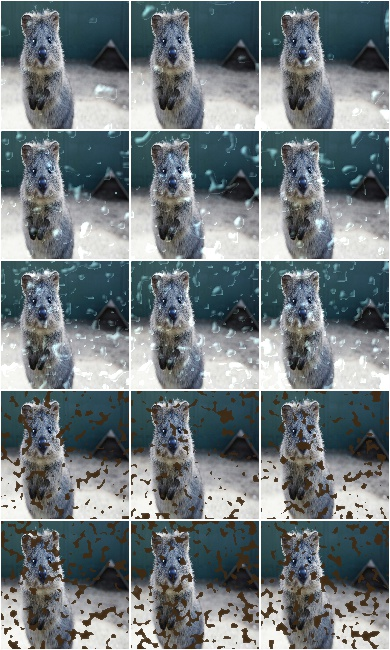Example. Create an augmenter around `spatter()`. Apply it to images using e.g. `aug(images=[image1, image2, ...])`:

```import imgaug.augmenters as iaa
aug = iaa.imgcorruptlike.Spatter(severity=2)
```

## Contrast¶

Wrapper around `contrast()`.

Note

This augmenter only affects images. Other data is not changed.

API link: `Contrast`

The image below visualizes severities `1` to `5` – one severity per row: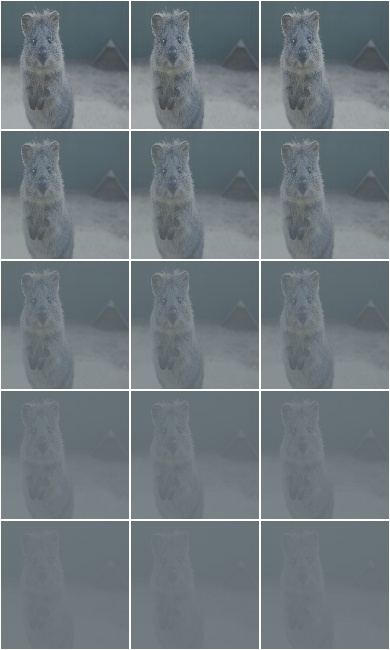Example. Create an augmenter around `contrast()`. Apply it to images using e.g. `aug(images=[image1, image2, ...])`:

```import imgaug.augmenters as iaa
aug = iaa.imgcorruptlike.Contrast(severity=2)
```

## Brightness¶

Wrapper around `brightness()`.

Note

This augmenter only affects images. Other data is not changed.

API link: `Brightness`

The image below visualizes severities `1` to `5` – one severity per row: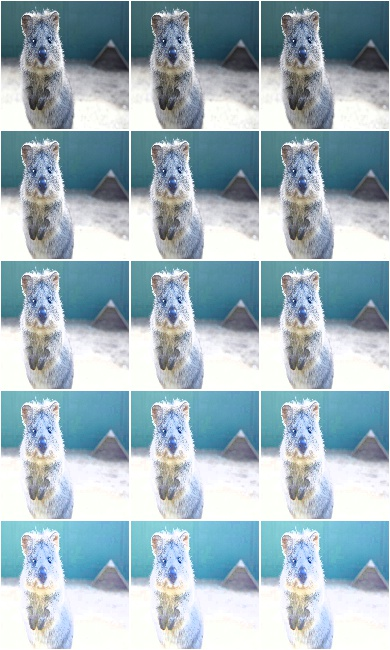Example. Create an augmenter around `brightness()`. Apply it to images using e.g. `aug(images=[image1, image2, ...])`:

```import imgaug.augmenters as iaa
aug = iaa.imgcorruptlike.Brightness(severity=2)
```

## Saturate¶

Wrapper around `saturate()`.

Note

This augmenter only affects images. Other data is not changed.

API link: `Saturate`

The image below visualizes severities `1` to `5` – one severity per row: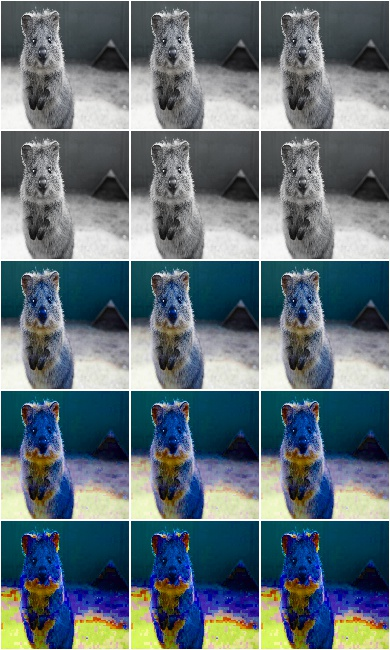Example. Create an augmenter around `saturate()`. Apply it to images using e.g. `aug(images=[image1, image2, ...])`:

```import imgaug.augmenters as iaa
aug = iaa.imgcorruptlike.Saturate(severity=2)
```

## JpegCompression¶

Wrapper around `jpeg_compression()`.

Note

This augmenter only affects images. Other data is not changed.

API link: `JpegCompression`

The image below visualizes severities `1` to `5` – one severity per row: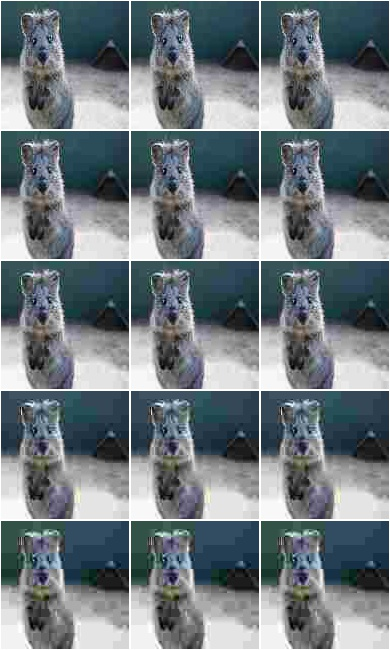Example. Create an augmenter around `jpeg_compression()`. Apply it to images using e.g. `aug(images=[image1, image2, ...])`:

```import imgaug.augmenters as iaa
aug = iaa.imgcorruptlike.JpegCompression(severity=2)
```

## Pixelate¶

Wrapper around `jpeg_compression()`.

Note

This augmenter only affects images. Other data is not changed.

Wrapper around `pixelate()`.

Note

This augmenter only affects images. Other data is not changed.

API link: `Pixelate`

The image below visualizes severities `1` to `5` – one severity per row: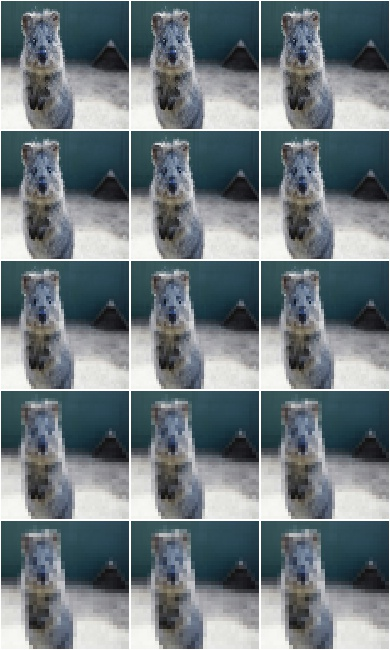Example. Create an augmenter around `pixelate()`. Apply it to images using e.g. `aug(images=[image1, image2, ...])`:

```import imgaug.augmenters as iaa
aug = iaa.imgcorruptlike.Pixelate(severity=2)
```

## ElasticTransform¶

Wrapper around `elastic_transform()`.

Note

This augmenter only affects images. Other data is not changed.

API link: `ElasticTransform`

The image below visualizes severities `1` to `5` – one severity per row: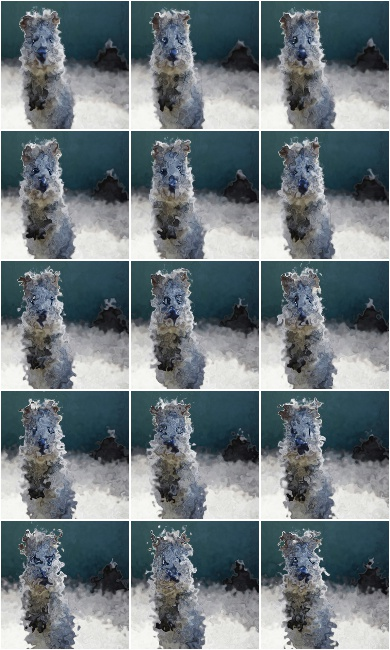Example. Create an augmenter around `elastic_transform()`. Apply it to images using e.g. `aug(images=[image1, image2, ...])`:

```import imgaug.augmenters as iaa
aug = iaa.imgcorruptlike.ElasticTransform(severity=2)
```